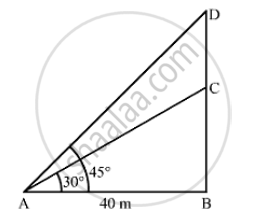# From a Point on the Ground 40m Away from the Foot of a Tower, the Angle of Elevation of the Top of the Tower is 30 . Find (I) the Height of the Tower, (Ii) the Depth of the Tank. - Mathematics

From a point on the ground 40m away from the foot of a tower, the angle of elevation of the top of the tower is 30 . The angle of elevation of the top of a water tank (on the top of the tower) is 45 , Find (i) the height of the tower, (ii) the depth of the tank.

#### SolutionLet BC be the tower and CD be the water tank.
We have,
AB  = 40m, ∠BAC = 30°  and ∠BAD =  45°
In ΔABD,

 tan 45° = (BD)/(AB)

⇒ 1 = (BD)/40

⇒ BD = 40 M

Now, in  Δ ABC

tan  30° = (BC)/(AB)

⇒1/ sqrt(3) = (BC) /40

⇒ BC = 40/sqrt(3)

⇒ BC = 40/ sqrt(3) xx sqrt(3)/sqrt(3)

⇒ BC = (40sqrt(3) )/3 m

"(i) The height of the tower," BC = (40 sqrt(3))/3 = (40 xx 1.73)/3 = 23.067  ~~ 23.1 m

"(ii) The depth of the tank " CD = (BD - BC) = (40-23.1 ) = 16.9 m

Concept: Heights and Distances
Is there an error in this question or solution?
Chapter 14: Height and Distance - Exercises

#### APPEARS IN

RS Aggarwal Secondary School Class 10 Maths
Chapter 14 Height and Distance
Exercises | Q 6

Share